## SGANNO Overwhelms SGPLOT

Updated: The following MWE better shows my situation.

``````data have;
input id :\$16. @@;
f=compress("https://fred.stlouisfed.org/graph/fredgraph.csv?id="||id);
infile _ url filevar=f firstobs=2 dsd truncover end=e;
do until(e);
input date yymmdd10. +1 value;
if value>. then output;
end;
cards;
will5000indfc indpro sp500 unrate dexuseu cpiaucsl gdp dgs10 fedfunds
;

data sganno(drop=v);
display="fill";
fillcolor="black";
filltransparency=0.8;
x1space="datavalue";
y1space="wallpercent";
function="polygon ";
infile "https://fred.stlouisfed.org/graph/fredgraph.csv?id=usrecm" url firstobs=2 dsd truncover;
input x1 yymmdd10. +1 v;
if v then do;
y1=0;
output;
function="polycont";
y1+100;
output;
x1=intnx("month",x1,1);
output;
y1+-100;
output;
end;
run;

ods html close;
ods results=off;
ods listing gpath="!userprofile\desktop\";
ods graphics/reset;

proc sgplot data=have sganno=sganno;
by notsorted id;
series x=date y=value;
xaxis valuesformat=year4.;
yaxis valuesformat=best8. type=log;
run;``````

I cannot simply adjust the SGANNO data set. How can I avoid the overwhelming SGANNO shades automatically?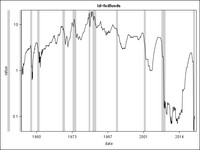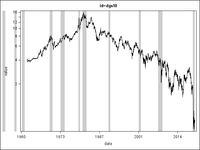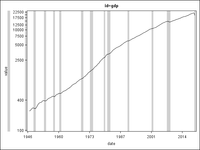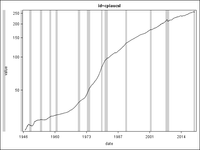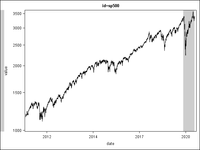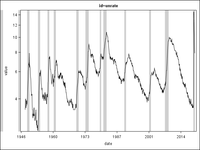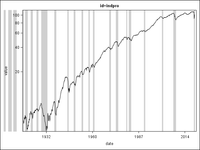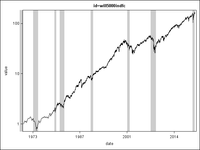Original: This MWE draws a shaded SGPLOT SERIES.

``````data data;
do t=1 to 100;
x+rannor(1);
output;
end;
run;

data sganno;
display="fill";
fillcolor="black";
filltransparency=0.8;
x1space="datavalue";
y1space="wallpercent";
do x1=-10 to 90 by 10;
function="polygon ";
y1=0;
output;
function="polycont";
y1=100;
output;
x1+10;
output;
y1=0;
output;
end;
run;

ods html close;
ods results=off;
ods listing gpath="!userprofile\desktop\";
ods graphics/reset imagename="sgplot";

proc sgplot data=data sganno=sganno;
series x=t y=x;
run;``````

SGANNO has six uniform shades starting at X1=-10, 10, 30, 50, 70, 90, so SGPLOT locates the first shade outside the box.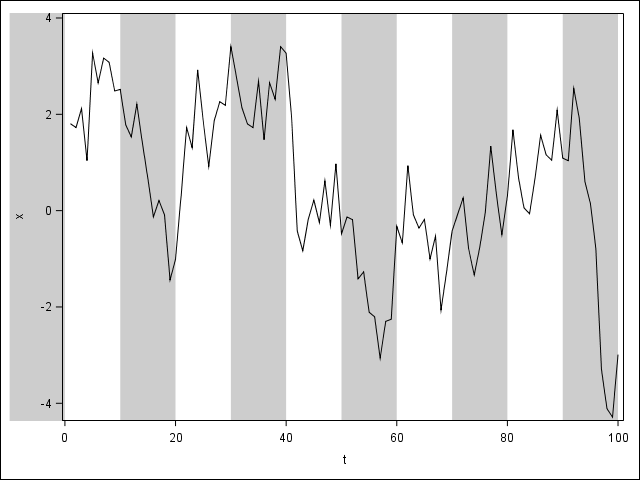I have manually used PROC SGPLOT DATA=DATA SGANNO=SGANNO(WHERE=(X1>=0)); to avoid this, but this requires too many micromanagements. How can I automatically limit SGANNO not to overwhelm the box?

1 ACCEPTED SOLUTION

Accepted Solutions

## Re: SGANNO Overwhelms SGPLOT

I think the BLOCK plot will work for what you're trying to do.  I used your value of t to make a modular variable (1 or 0).  I use this to make different blocks in the block plot, then alternate between black and white for the two colors.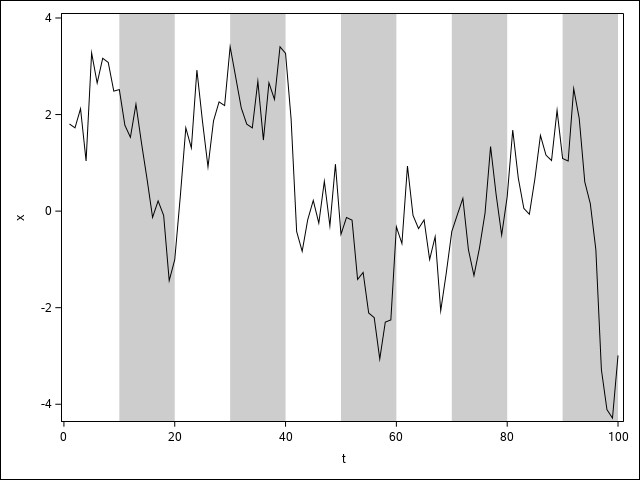``````data data;
do t=1 to 100;
x+rannor(1);
block=mod(floor(t/10),2);
output;
end;
run;

ods results;
ods graphics/reset imagename="sgplot";

proc sgplot data=data;
block x=t block=block / nooutline filltype=alternate
fillattrs=(color=white) altfillattrs=(color=black transparency=0.8)
nolabel novalues;
series x=t y=x;
run;
``````
18 REPLIES 18

## Re: SGANNO Overwhelms SGPLOT

Is there a particular reason to implement this using SGANNO?

Try using a Band or Polygon statement instead?
Or possibly even the colorbands option on the xaxis/yaxis statement? I feel like those would align better if your data changes..

## Re: SGANNO Overwhelms SGPLOT

No specific reason—I have been using SGANNO just following How to show recessions on your SGplot line graph.

## Re: SGANNO Overwhelms SGPLOT

Well, annotation allows you to control your features but that means you lose some of the automatic options. More control means you have to specify the details....I'd suggest switching to a different approach that was more dynamic if you need to do it more than just as a single post.

I've moved the post to the Graphics forum, perhaps one of the SAS graphing guru's may have a better answer for you 🙂

## Re: SGANNO Overwhelms SGPLOT

I think the BLOCK plot will work for what you're trying to do.  I used your value of t to make a modular variable (1 or 0).  I use this to make different blocks in the block plot, then alternate between black and white for the two colors.``````data data;
do t=1 to 100;
x+rannor(1);
block=mod(floor(t/10),2);
output;
end;
run;

ods results;
ods graphics/reset imagename="sgplot";

proc sgplot data=data;
block x=t block=block / nooutline filltype=alternate
fillattrs=(color=white) altfillattrs=(color=black transparency=0.8)
nolabel novalues;
series x=t y=x;
run;
``````

## Re: SGANNO Overwhelms SGPLOT

@DanH_sas Not to hijack this thread, but is there a reason why there's no Y-axis variant of block plot? I've actually wanted something like that quite a few times.DanH_sas
SAS Super FREQ

## Re: SGANNO Overwhelms SGPLOT

@JeffMeyers , we have not had a lot of call for it. There are workarounds (using Band plots, etc.); but, if you need this support, I would suggest putting in a request with Technical Support so that we can track it.

Thanks!

DanPGStats
Opal | Level 21

## Re: SGANNO Overwhelms SGPLOT

Adding two macro variables could provide some automation:

``````data data;
do t=1 to 100;
x+rannor(1);
output;
end;
run;

proc sql;
select 10*floor(min(t)/10), 10*ceil(max(t)/10) into :t0, :t1
from data;
quit;

data sganno;
display="fill";
fillcolor="black";
filltransparency=0.8;
x1space="datavalue";
y1space="wallpercent";
do x1=&t0 to &t1-10 by 10;
function="polygon ";
y1=0;
output;
function="polycont";
y1=100;
output;
x1+10;
output;
y1=0;
output;
end;
run;

proc sgplot data=data sganno=sganno;
series x=t y=x;
run;``````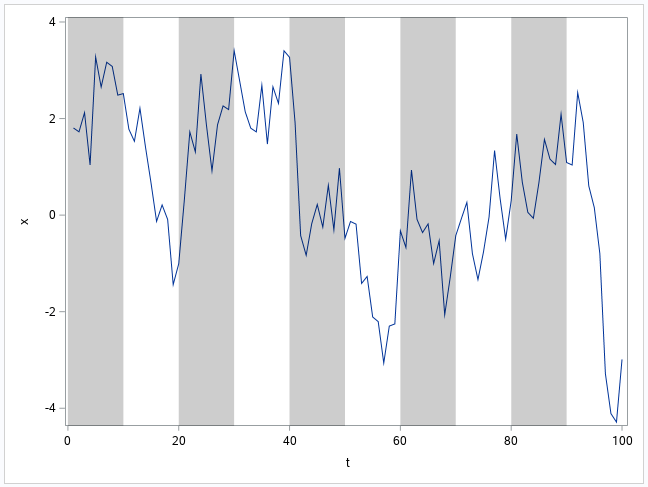PG

## Re: SGANNO Overwhelms SGPLOT

The original code was just an MWE. I know that I can change the SGANNO data set to plot properly but, as described below, wondered if SGPLOT has an automatic solution to avoid the overwhelming shades.

## Re: SGANNO Overwhelms SGPLOT

Why are you starting your x1 values for the annotated shaded bars at -10?

`do x1=-10 to 90 by 10;`

Since your x axis starts at 0, then the -10 is going to be outside the axes. If you start at 0 or 10, I think you will get something more of what you're looking for!

## Re: SGANNO Overwhelms SGPLOT

That was just an MWE. My real data are more similar to this.

``````data have;
input id :\$16. @@;
f=compress("https://fred.stlouisfed.org/graph/fredgraph.csv?id="||id);
infile _ url filevar=f firstobs=2 dsd truncover end=e;
do until(e);
input date yymmdd10. +1 value;
if value>. then output;
end;
cards;
will5000indfc indpro sp500 unrate dexuseu cpiaucsl gdp dgs10 fedfunds
;

data sganno(drop=v);
display="fill";
fillcolor="black";
filltransparency=0.8;
x1space="datavalue";
y1space="wallpercent";
function="polygon ";
infile "https://fred.stlouisfed.org/graph/fredgraph.csv?id=usrecm" url firstobs=2 dsd truncover;
input x1 yymmdd10. +1 v;
if v then do;
y1=0;
output;
function="polycont";
y1+100;
output;
x1=intnx("month",x1,1);
output;
y1+-100;
output;
end;
run;

ods html close;
ods results=off;
ods listing gpath="!userprofile\desktop\";
ods graphics/reset;

proc sgplot data=have sganno=sganno;
by notsorted id;
series x=date y=value;
xaxis valuesformat=year4.;
yaxis valuesformat=best8. type=log;
run;``````

I have multiple time series to plot with one SGANNO data set shared by the time series. That is why I cannot simply adjust X1 for each.Hope this better explains.

## Re: SGANNO Overwhelms SGPLOT

I'm a bit confused by what you're trying to do.  Do you want the shading to alternate along a specific length of time, or just every 10% of the graph?

If it's by a specific length of time then my solution would work.  If it's by 10% of the graph then you would want to annotate by wall percent instead of by data value on your x-axis.

## Re: SGANNO Overwhelms SGPLOT

I am not randomly shading 10% of each graph. NBER based Recession Indicators for the United States from the Peak through the Trough is a dummy time series that is 1 if the market is in recession.This monthly series is from December 1854 to September 2020, and I am shading the plots of other multiple time series variables such as Industrial Production: Total Index (from January 1919) and Unemployment Rate (from January 1948) as follows.The problem is that the time series variables I am plotting start later (for example, January 1919) than the recession series (December 1854) I am shading, and the SGPLOT SGANNO unexpectedly spans the ranges outside the plotting area. I don't want to apply the shades outside the box of the time series plots.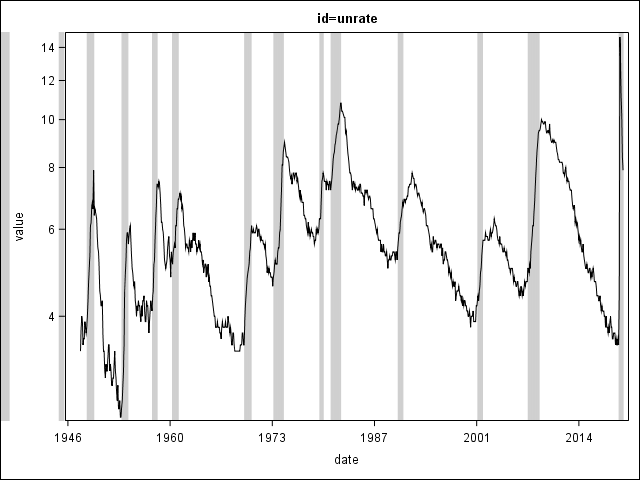This is the same Unemployment Rate series drawn by SGPLOT. The SGANNO shades 1937, 1938, and 1945 because the market was in recession, but the Unemployment Rate series starts in January 1948, so the two pre-1948 shades are making the outcome ugly.

## Re: SGANNO Overwhelms SGPLOT

I would then suggest looking at my earlier example using the BLOCK plot.  If you have or can calculate a variable that is equal to 1 whenever you want it shaded and can make it 0 when you don't want it shaded, you can use the BLOCK plot to alternate the shading like in my example.  Then SGPLOT would automatically limit where the shading happens (it won't draw it outside the axes like annotation does).  I don't have your exact data to give a more specific example.

## Re: SGANNO Overwhelms SGPLOT

So the corresponding MWE will be this.

``````data have;
n=_n_;
input id :\$16. @@;
f=compress("https://fred.stlouisfed.org/graph/fredgraph.csv?id="||id);
infile _ url filevar=f firstobs=2 dsd truncover end=e;
do until(e);
input date yymmdd10. +1 value;
if value>. then output;
end;
format date yymmdd10.;
cards;
will5000indfc indpro sp500 unrate dexuseu cpiaucsl gdp dgs10 fedfunds usrecm
;

proc sql;
create table want(drop=n) as
select i.*,j.value as usrecm
from have(where=(id^="usrecm")) i
left join have(where=(id="usrecm")) j
on put(i.date,yymm.)=put(j.date,yymm.)
order by i.n,date;
quit;

ods html close;
ods results=off;
ods listing gpath="!userprofile\desktop\";
ods graphics/reset;

proc sgplot;
by notsorted id;
series x=date y=value;
block x=date block=usrecm/nooutline filltype=alternate fillattrs=(transparency=1) altfillattrs=(color=black transparency=0.8) nolabel;
xaxis valuesformat=year4. values=("1jan1901"d to "31dec2100"d by year10) valueshint;
yaxis valuesformat=best8. type=log;
run;``````

I saw the BLOCK statement for the first time because I have been shading using SGANNO following How to show recessions on your SGplot line graph as aforementioned. It seems this is simpler. But may I ask two more questions?1. The BLOCK FILLATTRS ALTFILLATTRS results depend on the first value of the dummy variable for BLOCK. For example, the shading outcome is reversed here because the dummy variable value at the starting point of the series is 1 here. How can I correct this?2. I often use multiple shading colors and their orders are not always consistent (for example, white-red-green-red-white-red-green, etc.). Can I use this BLOCK solution for this case too?

Discussion stats
• 18 replies
• 1337 views
• 15 likes
• 6 in conversation